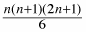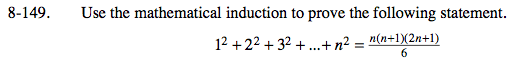### Home > PC > Chapter 8 > Lesson 8.2.6 > Problem8-149

8-149.

Use the mathematical induction to prove the following statement. Homework Help ✎

12 + 22 + 32 + … + n2 =12 + ... + k2 + (k + 1)2 =

So the induction step is true.

Since we know the statement is true for n = 1 and the induction step is true, we know that the statement is true for all positive integers n.